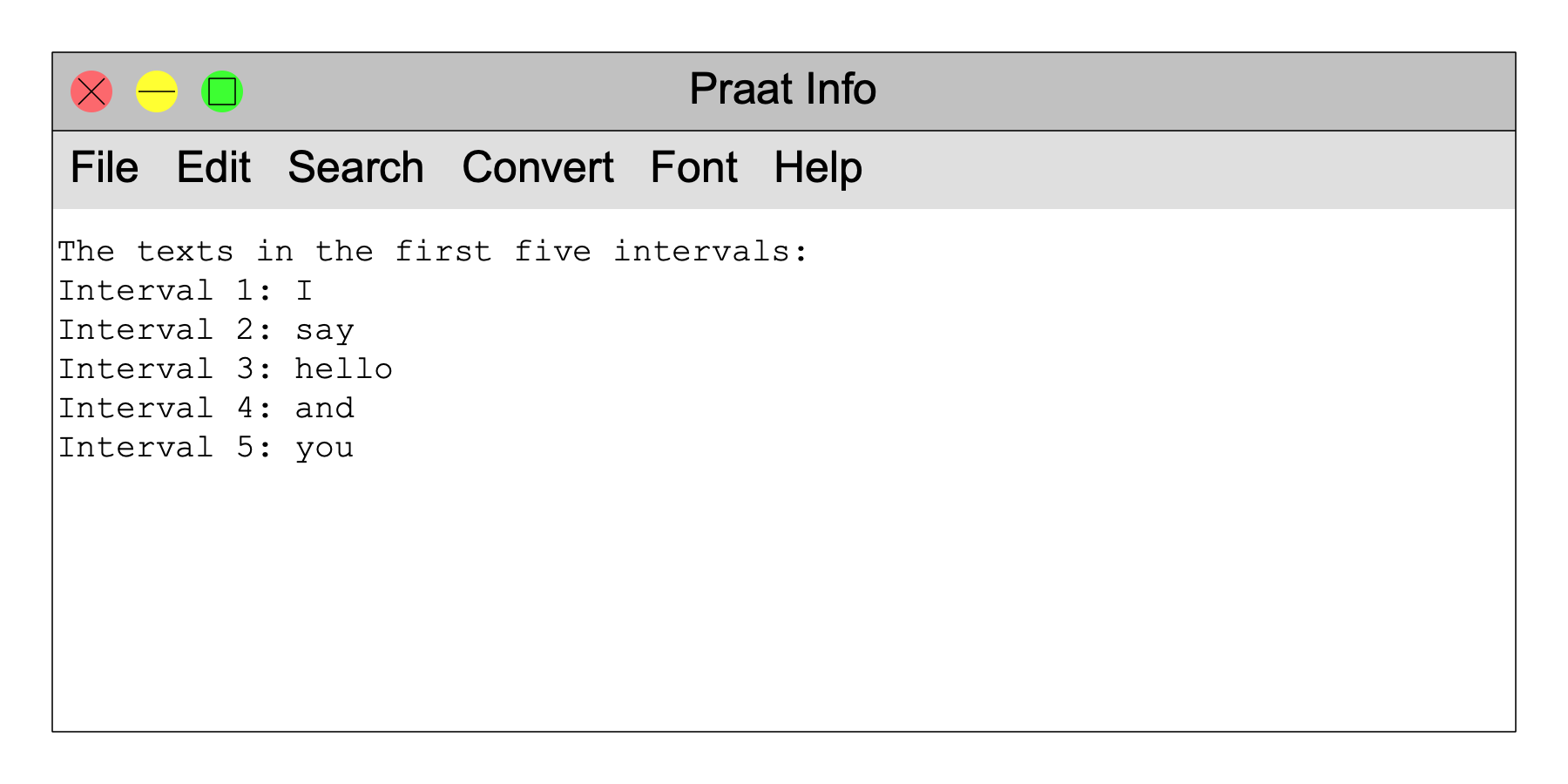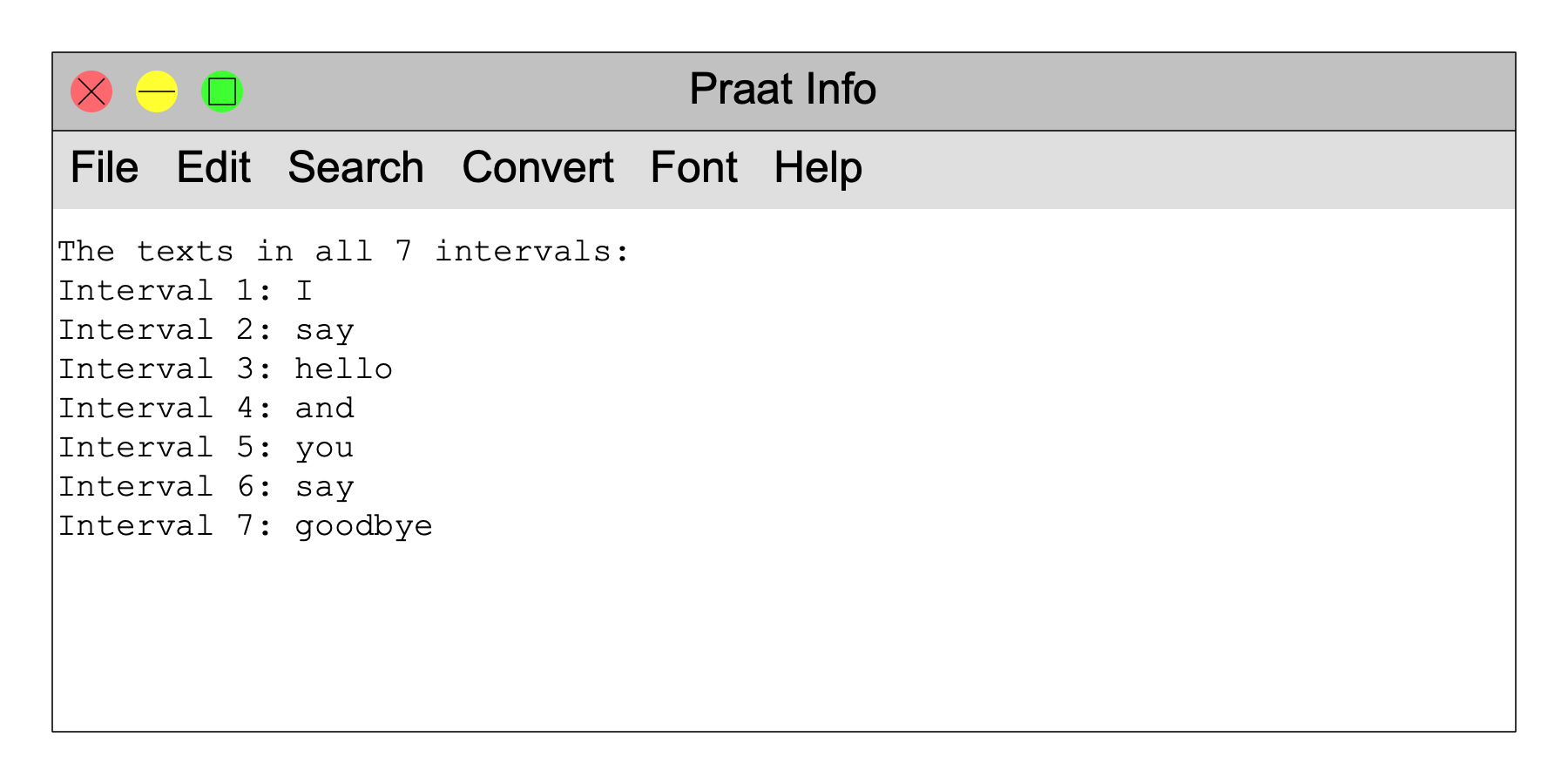Scripting 3.6. “For” loops

The power of a procedural programming language is most easily illustrated with the for-loop.

Take the example of the previous page, whereas you wanted to know the text in the third interval of the first tier of a selected TextGrid. It’s easy to imagine that you actually want the texts of all the first five intervals. With knowledge from the previous sections, you could write it like this:

```    writeInfoLine: “The texts in the first five intervals:”``` ```    text\$ = Get label of interval: 1, 1``` ```    appendInfoLine: “Interval 1: ”, text\$``` ```    text\$ = Get label of interval: 1, 2``` ```    appendInfoLine: “Interval 2: ”, text\$``` ```    text\$ = Get label of interval: 1, 3``` ```    appendInfoLine: “Interval 3: ”, text\$``` ```    text\$ = Get label of interval: 1, 4``` ```    appendInfoLine: “Interval 4: ”, text\$``` ```    text\$ = Get label of interval: 1, 5``` ```    appendInfoLine: “Interval 5: ”, text\$```

The result will be something likeThis can be done more nicely. The first step is to realize that the sentences starting with `text\$` are similar to each other, and the sentence starting with `appendInfoLine` are also similar to each other. They only differ in the interval number, and can therefore be made identical by using a variable for the interval number, like this:

```    writeInfoLine: “The texts in the first five intervals:”``` ```    intervalNumber = 1``` ```    text\$ = Get label of interval: 1, intervalNumber``` ```    appendInfoLine: “Interval ”, intervalNumber, “: ”, text\$``` ```    intervalNumber = 2``` ```    text\$ = Get label of interval: 1, intervalNumber``` ```    appendInfoLine: “Interval ”, intervalNumber, “: ”, text\$``` ```    intervalNumber = 3``` ```    text\$ = Get label of interval: 1, intervalNumber``` ```    appendInfoLine: “Interval ”, intervalNumber, “: ”, text\$``` ```    intervalNumber = 4``` ```    text\$ = Get label of interval: 1, intervalNumber``` ```    appendInfoLine: “Interval ”, intervalNumber, “: ”, text\$``` ```    intervalNumber = 5``` ```    text\$ = Get label of interval: 1, intervalNumber``` ```    appendInfoLine: “Interval ”, intervalNumber, “: ”, text\$```

A new trick that you see here is that as a numeric argument (Interval number, the second argument to Get label of interval...), you can use not only a number (as in all previous examples), but also a variable (`intervalNumber`). The rest of the script should be known stuff by now.

The script above is long, but it can be made much shorter with the use of a for-loop:

```    writeInfoLine: “The texts in the first five intervals:”``` ```    for intervalNumber from 1 to 5``` ```       text\$ = Get label of interval: 1, intervalNumber``` ```       appendInfoLine: “Interval ”, intervalNumber, “: ”, text\$``` ```    endfor```

The two lines that were repeated five times in the previous version now show up with indentation between a `for` line and its corresponding `endfor`. Those two lines (the `text\$` and the `appendInfoLine` line) are executed five times: for `intervalNumber` equal to 1, for `intervalNumber` equal to 2, for `intervalNumber` equal to 3, for `intervalNumber` equal to 4, and for `intervalNumber` equal to 5, in that order.

In the above example, using a loop does not do much more than save eight lines, at the cost of adding two new lines. But imagine the case in which you want to list all the texts in the intervals: the version without the loop is no longer possible. By contrast, the version with the loop is still possible, because we have the command Get number of intervals..., which gives us the number of intervals in the specified tier (here, tier 1). So you do:

```    numberOfIntervals = Get number of intervals: 1``` ```    writeInfoLine: “The texts in all ”, numberOfIntervals, “ intervals:”``` ```    for intervalNumber from 1 to numberOfIntervals``` ```       text\$ = Get label of interval: 1, intervalNumber``` ```       appendInfoLine: “Interval ”, intervalNumber, “: ”, text\$``` ```    endfor```

This may yield something likeThis is the first script in this tutorial that is useful in itself. On the basis of it you can create all kinds of ways to list the texts in intervals. Here is how you would also list the durations of those intervals:

```    numberOfIntervals = Get number of intervals: 1``` ```    writeInfoLine: “The durations and texts in all ”, numberOfIntervals, “ intervals:”``` ```    for intervalNumber from 1 to numberOfIntervals``` ```       startTime = Get start point: 1, intervalNumber``` ```       endTime = Get end point: 1, intervalNumber``` ```       duration = endTime - startTime``` ```       text\$ = Get label of interval: 1, intervalNumber``` ```       appendInfoLine: “Interval ”, intervalNumber, “ is ”, duration, “ seconds long and contains the text: ”, text\$``` ```    endfor```